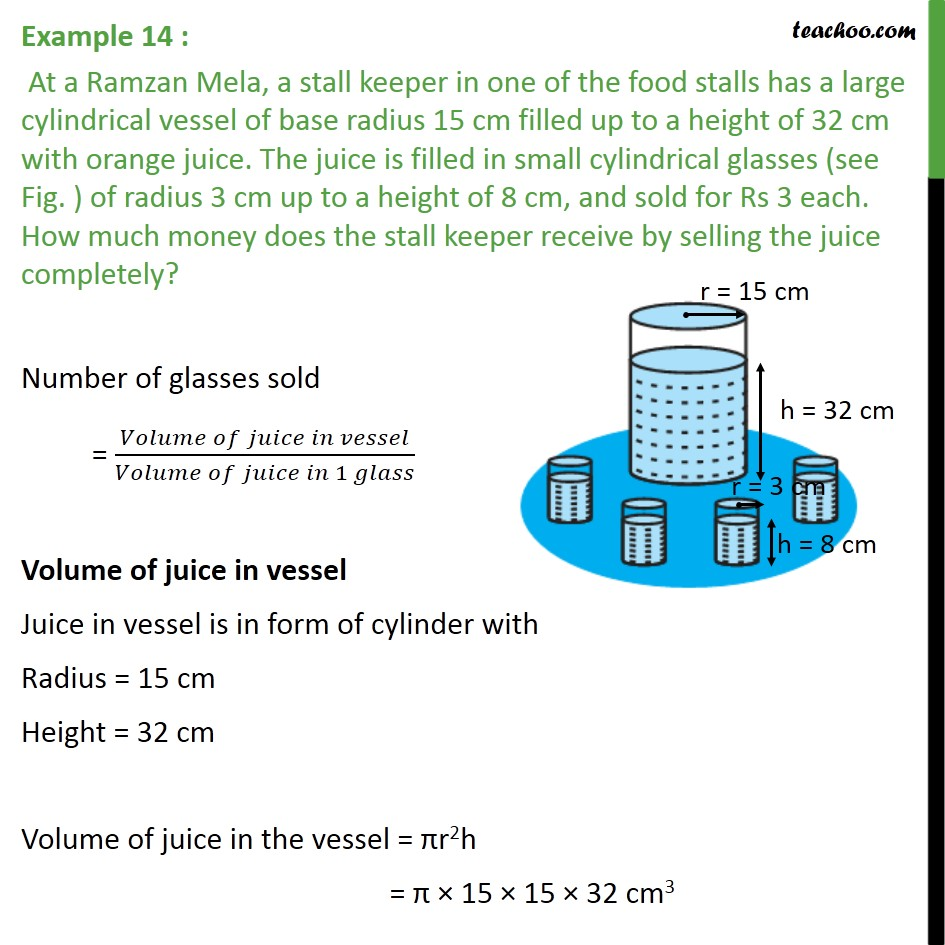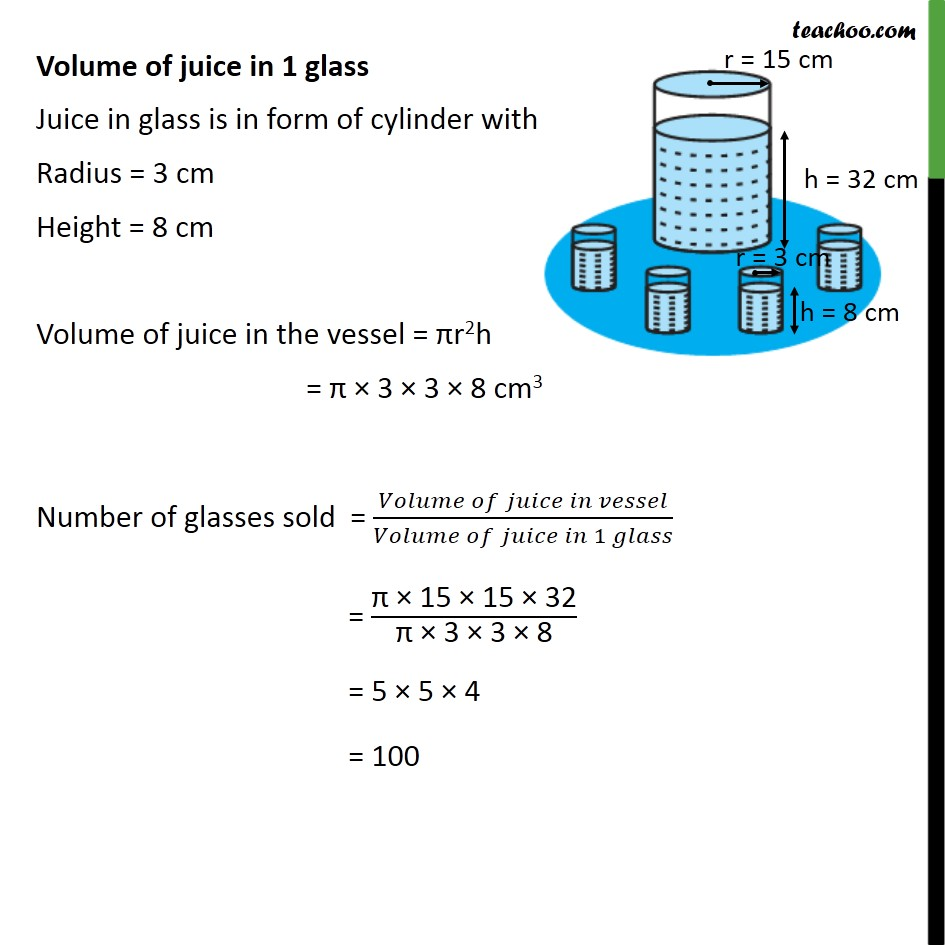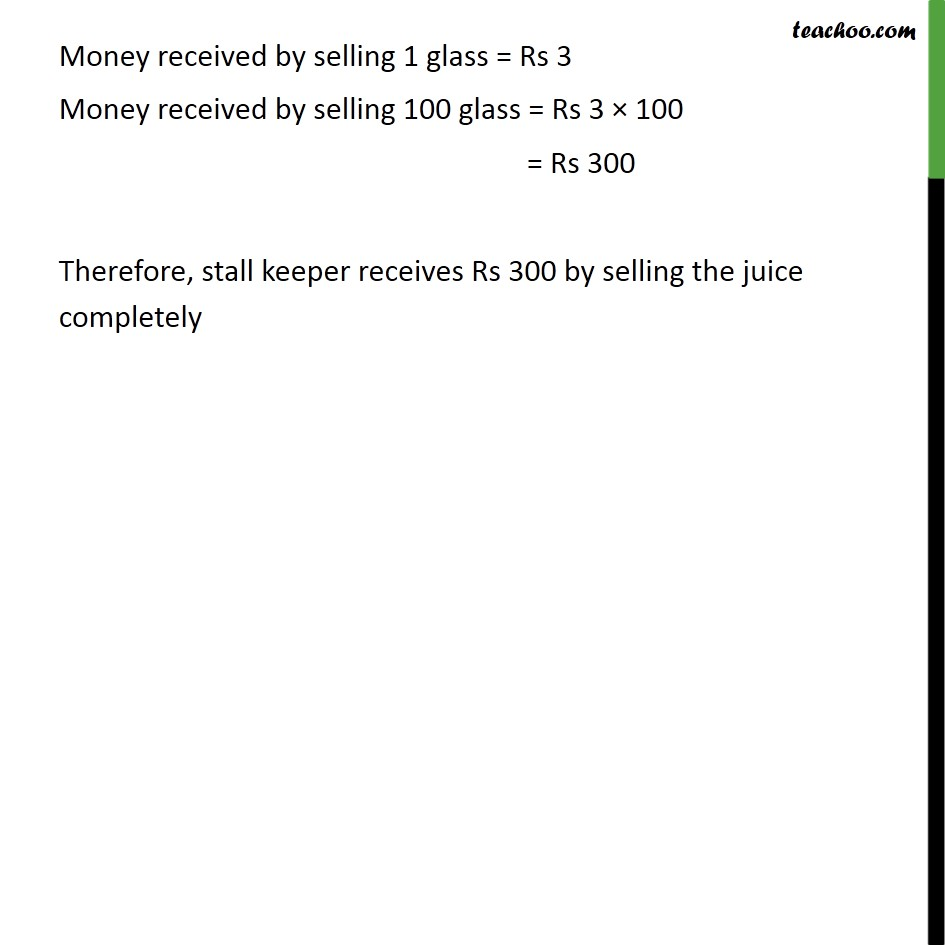1. Chapter 13 Class 9 Surface Areas and Volumes (Term 2)
2. Serial order wise
3. Examples

Transcript

Example 14 : At a Ramzan Mela, a stall keeper in one of the food stalls has a large cylindrical vessel of base radius 15 cm filled up to a height of 32 cm with orange juice. The juice is filled in small cylindrical glasses (see Fig. ) of radius 3 cm up to a height of 8 cm, and sold for Rs 3 each. How much money does the stall keeper receive by selling the juice completely? Number of glasses sold = ( )/( 1 ) Volume of juice in vessel Juice in vessel is in form of cylinder with Radius = 15 cm Height = 32 cm Volume of juice in the vessel = r2h = 15 15 32 cm3 Volume of juice in 1 glass Juice in glass is in form of cylinder with Radius = 3 cm Height = 8 cm Volume of juice in the vessel = r2h = 3 3 8 cm3 Number of glasses sold = ( )/( 1 ) = " 15 15 32" /" 3 3 8" = 5 5 4 = 100 Money received by selling 1 glass = Rs 3 Money received by selling 100 glass = Rs 3 100 = Rs 300 Therefore, stall keeper receives Rs 300 by selling the juice completely